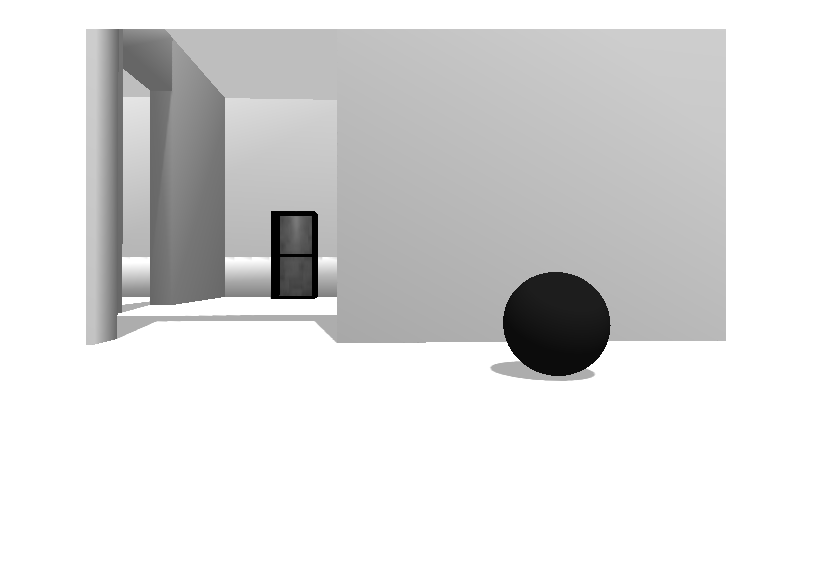## Execute Code Based on ROS Time

Using a `rosrate` object allows you to control the rate of your code execution based on the ROS Time `/clock` topic or system time on your computer. By executing code at constant intervals, you can accurately time and schedule tasks. These examples show different applications for the `rosrate` object including its uses with ROS image messages and sending commands for robot control.

For other applications based on system time, consider the `rateControl` (Navigation Toolbox) object.

### Send Fixed-rate Control Commands To A Robot

This example shows to send regular commands to a robot at a fixed rate. It uses the `Rate` object to execute a loop that publishes `std_msgs/Twist` messages to the network at a regular interval.

Setup ROS network. Specify the IP address if your ROS network already exists.

`rosinit`
```Launching ROS Core... Done in 0.40404 seconds. Initializing ROS master on http://172.29.205.18:50504. Initializing global node /matlab_global_node_87393 with NodeURI http://dcc433567glnxa64:33399/ and MasterURI http://localhost:50504. ```

Setup publisher and message to send `Twist` commands.

```[pub,msg] = rospublisher('/cmd_vel','geometry_msgs/Twist'); msg.Linear.X = 0.5; msg.Angular.Z = -0.5;```

Create `Rate` object with specified loop parameters.

```desiredRate = 10; rate = robotics.Rate(desiredRate); rate.OverrunAction = 'drop'```
```rate = rateControl with properties: DesiredRate: 10 DesiredPeriod: 0.1000 OverrunAction: 'drop' TotalElapsedTime: 0.1791 LastPeriod: NaN ```

Run loop and hold each iteration using `waitfor(rate)`. Send the `Twist` message inside the loop. Reset the `Rate` object before the loop to reset timing.

```reset(rate) while rate.TotalElapsedTime < 10 send(pub,msg) waitfor(rate); end```

View statistics of fixed-rate execution. Look at `AveragePeriod` to verify the desired rate was maintained.

`statistics(rate)`
```ans = struct with fields: Periods: [0.1013 0.0988 0.1000 0.1001 0.0999 0.1000 0.1000 ... ] NumPeriods: 100 AveragePeriod: 0.1000 StandardDeviation: 1.8835e-04 NumOverruns: 0 ```

Shut down ROS network.

`rosshutdown`
```Shutting down global node /matlab_global_node_87393 with NodeURI http://dcc433567glnxa64:33399/ and MasterURI http://localhost:50504. Shutting down ROS master on http://172.29.205.18:50504. ```

### Fixed-rate Publishing of ROS Image Data

This example shows how to regularly publish and receive image messages using ROS and the `rosrate` function. The `rosrate` function creates a `Rate` object to regularly access the `/camera/rgb/image_raw` topic on the ROS network using a subscriber. The rgb image is converted to a grayscale using `rgb2gray` and republished at a regular interval. Parameters such as the IP address and topic names vary with your robot and setup.

Connect to ROS network. Setup subscriber, publisher, and data message.

```ipaddress = '192.168.203.129'; % Replace with your network address rosinit(ipaddress)```
```Initializing global node /matlab_global_node_10650 with NodeURI http://192.168.203.1:50899/ ```
```sub = rossubscriber('/camera/rgb/image_raw'); pub = rospublisher('/camera/gray/image_gray','sensor_msgs/Image'); msgGray = rosmessage('sensor_msgs/Image'); msgGray.Encoding = 'mono8';```

Receive the first image message. Extract image and convert to a grayscale image. Display grayscale image and publish the message.

```msgImg = receive(sub); img = readImage(msgImg); grayImg = rgb2gray(img); imshow(grayImg)``````writeImage(msgGray,grayImg) send(pub,msgGray)```

Create ROS `Rate` object to execute at 10 Hz. Set a loop time and the `OverrunAction` for handling

```desiredRate = 10; loopTime = 5; overrunAction = 'slip'; rate = rosrate(desiredRate); r.OverrunAction = overrunAction;```

Begin loop to receive, process and send messages every 0.1 seconds (10 Hz). Reset the `Rate` object before beginning.

```reset(rate) for i = 1:desiredRate*loopTime msgImg = receive(sub); img = readImage(msgImg); grayImg = rgb2gray(img); writeImage(msgGray,grayImg) send(pub,msgGray) waitfor(rate); end```

View the statistics for the code execution. `AveragePeriod` and `StandardDeviation` show how well the code maintained the `desiredRate`. `OverRuns` occur when data processing takes longer than the desired period length.

`statistics(rate)`
```ans = struct with fields: Periods: [1×50 double] NumPeriods: 50 AveragePeriod: 0.1000 StandardDeviation: 0.0083 NumOverruns: 0 ```

Shut down ROS node

`rosshutdown`
```Shutting down global node /matlab_global_node_10650 with NodeURI http://192.168.203.1:50899/ ```